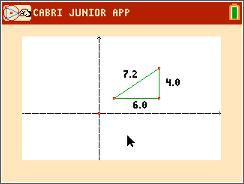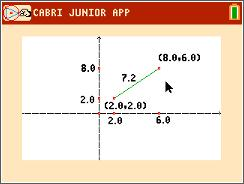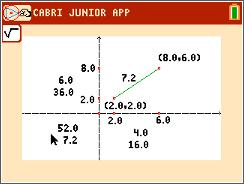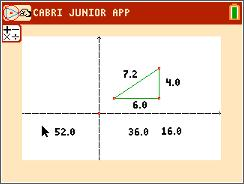# Activities

••• ##### Subject Area

• Math: Geometry: Analytic Geometry

• ##### Author9-12

45 Minutes

• ##### Device
• TI-84 Plus
• TI-84 Plus Silver Edition
•TI-84 Plus C Silver Edition
•TI-84 Plus CE
• ##### Software

TI Connect™ CE

## Distances in the Coordinate Plane#### Activity Overview

Students will explore distances in the coordinate plane. After finding the coordinates of a segment’s endpoints, students will substitute these values into the distance formula and compare the results to the measured length of the segment. Then students will find the distance between the endpoints using the Pythagorean Theorem.

#### Key Steps

•Students construct a segment and find the coordinates of the endpoints.  They use the D. & Length tool to find length of the segment and distance from the endpoints to each axis.

•Then students will use the Calculate tool to find the length of the segment using the coordinates of the endpoints.

•In the second part of the activity, students calculate the length of a hypotenuse using the side length of a triangle.